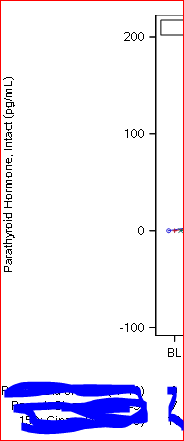data_null__

## SGPLOT position of Y axis label in relation to XAXISTABLE

SAS 9.4 (TS1M3) sun unix

My code

``````proc sgplot data=&plotdata;
series x=avisitn y=median / markers group=&trt groupdisplay=cluster name='legend';
highlow x=avisitn high=q3 low=q1 / group=&trt groupdisplay=cluster lowcap=serif highcap=serif;
xaxistable n / class=&trt;
keylegend 'legend' / autoitemsize location=inside  TITLE=" ";
xaxis values=(&visitlist);
label avisitn='Week' &trt=' ';
format &trt xtrt. avisitn avisitn.;
run;
``````

and the relevant part of the figure where you will notice that the long XAXISTABLE row labels appear to be influencing the location of the of the Y axis label.  I would like the Y axis label to move closer to the Y axis tick labels.  Can I do that?1 ACCEPTED SOLUTION

Accepted SolutionsJay54
Meteorite | Level 14

## Re: SGPLOT position of Y axis label in relation to XAXISTABLE

I too have encountered this case.  Using SGPLOT with OUTER axis table that has long class labels pushes the Y axis label out.  This is due to the internal uniform settings in the LAYOUT LATTICE that SGPLOT uses to layout the plots.

There are two ways to avoid this besides using Annotate.  One is to place the Subjects table INSIDE the graph using SGPLOT.  The other us to use GTL, suppress the Yaxis label, and draw your own using DRAWTEXT.  This is similar to Annotate, but a little more determinate.

``````/*--Define templage for Survival Plot--*/
proc template;
define statgraph Fig_8_7_Survival_plot_out;
begingraph  / axislineextent=data;
entrytitle 'Product-Limit Survival Estimates';
entrytitle 'With Number of AML Subjects at Risk' / textattrs=(size=8);
layout lattice / columns=1 columndatarange=union rowweights=preferred rowgutter=10px;
layout overlay / walldisplay=none
xaxisopts=(labelattrs=(size=8) tickvalueattrs=(size=7))
yaxisopts=(labelattrs=(size=8) tickvalueattrs=(size=7) display=(ticks tickvalues line));
stepplot x=time y=survival / group=stratum name='s';
scatterplot x=time y=censored / markerattrs=(symbol=plus) name='c';
scatterplot x=time y=censored / markerattrs=(symbol=plus) GROUP=stratum;
discretelegend 'c' / location=inside halign=right valign=top valueattrs=(size=7);
discretelegend 's' / valueattrs=(size=7);

/*--Draw the Y axis label closer to the axis--*/
drawtext textattrs=(size=8) 'Survival Probability' / x=-6 y=50 anchor=bottom
xspace=wallpercent yspace=wallpercent rotate=90 width=50;
endlayout;

layout overlay / walldisplay=none xaxisopts=(display=none);
/*--Subjects at risk--*/
axistable x=tatrisk value=atrisk / class=stratum colorgroup=stratum
labelattrs=(size=7) valueattrs=(size=7)
title='Subjects At Risk' titleattrs=(size=7);
endlayout;

endlayout;
endgraph;
end;
run;``````
2 REPLIES 2data_null__

## Re: SGPLOT position of Y axis label in relation to XAXISTABLE

I used SGANNO to annotate the label in the position I wanted it.  It is a bit fiddly because I have Y values on different figures with different number of digits in the Y axis tick labels.  Some have 2 some 4 so I have to adjust the X1 percent.  The code goes like this mostly copied from usage note sample program.

``````   data &sganno;
if 0 then set &data(keep=&var);
set &m._yrange(keep=ywidth);
retain function 'text' x1space 'wallpercent' y1space 'datapercent'
rotate 90 anchor "center" width 64;* textweight 'bold';
length label \$64;
label=vlabel(&var);
select(ywidth);
when(2)   x1=-4.5;
when(4)   x1=-6.5;
otherwise x1=-5.5;
end;
y1=50;
output;
stop;
run;``````

`yaxis display=(nolabel)`

to the SGPLOTJay54
Meteorite | Level 14

## Re: SGPLOT position of Y axis label in relation to XAXISTABLE

I too have encountered this case.  Using SGPLOT with OUTER axis table that has long class labels pushes the Y axis label out.  This is due to the internal uniform settings in the LAYOUT LATTICE that SGPLOT uses to layout the plots.

There are two ways to avoid this besides using Annotate.  One is to place the Subjects table INSIDE the graph using SGPLOT.  The other us to use GTL, suppress the Yaxis label, and draw your own using DRAWTEXT.  This is similar to Annotate, but a little more determinate.

``````/*--Define templage for Survival Plot--*/
proc template;
define statgraph Fig_8_7_Survival_plot_out;
begingraph  / axislineextent=data;
entrytitle 'Product-Limit Survival Estimates';
entrytitle 'With Number of AML Subjects at Risk' / textattrs=(size=8);
layout lattice / columns=1 columndatarange=union rowweights=preferred rowgutter=10px;
layout overlay / walldisplay=none
xaxisopts=(labelattrs=(size=8) tickvalueattrs=(size=7))
yaxisopts=(labelattrs=(size=8) tickvalueattrs=(size=7) display=(ticks tickvalues line));
stepplot x=time y=survival / group=stratum name='s';
scatterplot x=time y=censored / markerattrs=(symbol=plus) name='c';
scatterplot x=time y=censored / markerattrs=(symbol=plus) GROUP=stratum;
discretelegend 'c' / location=inside halign=right valign=top valueattrs=(size=7);
discretelegend 's' / valueattrs=(size=7);

/*--Draw the Y axis label closer to the axis--*/
drawtext textattrs=(size=8) 'Survival Probability' / x=-6 y=50 anchor=bottom
xspace=wallpercent yspace=wallpercent rotate=90 width=50;
endlayout;

layout overlay / walldisplay=none xaxisopts=(display=none);
/*--Subjects at risk--*/
axistable x=tatrisk value=atrisk / class=stratum colorgroup=stratum
labelattrs=(size=7) valueattrs=(size=7)
title='Subjects At Risk' titleattrs=(size=7);
endlayout;

endlayout;
endgraph;
end;
run;``````
Discussion stats
• 2 replies
• 3728 views
• 0 likes
• 2 in conversation